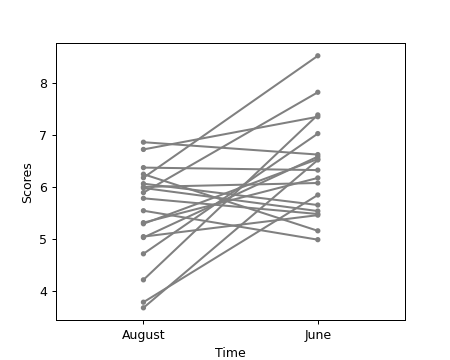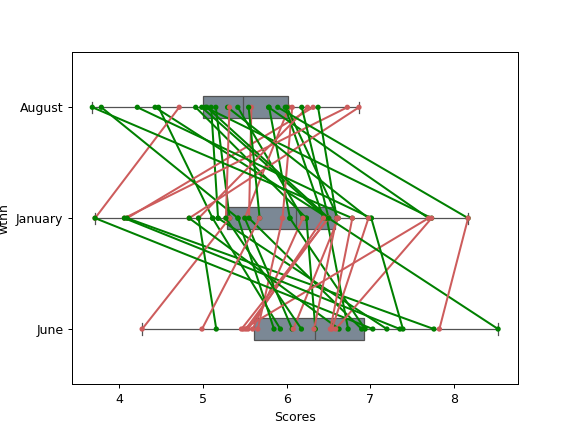# pingouin.plot_paired

pingouin.plot_paired(data=None, dv=None, within=None, subject=None, order=None, boxplot=True, boxplot_in_front=False, orient='v', figsize=4, 4, dpi=100, ax=None, colors=['green', 'grey', 'indianred'], pointplot_kwargs={'scale': 0.6, 'marker': '.'}, boxplot_kwargs={'color': 'lightslategrey', 'width': 0.2})[source]

Paired plot.

Parameters
datapandas.DataFrame

Long-format dataFrame.

dvstring

Name of column containing the dependent variable.

withinstring

Name of column containing the within-subject factor. Note that within must have exactly two within-subject levels (= two unique values).

subjectstring

Name of column containing the subject identifier.

orderlist of str

List of values in within that define the order of elements on the x-axis of the plot. If None, uses alphabetical order.

boxplotboolean

If True, add a boxplot to the paired lines using the seaborn.boxplot() function.

boxplot_in_frontboolean

If True, the boxplot is plotted on the foreground (i.e. above the individual lines) and with a slight transparency. This makes the overall plot more readable when plotting a large numbers of subjects.

New in version 0.3.8.

orientstring

Plot the boxplots vertically and the subjects on the x-axis if orient='v' (default). Set to orient='h' to rotate the plot by by 90 degrees.

New in version 0.3.9.

figsizetuple

Figsize in inches

dpiint

Resolution of the figure in dots per inches.

axmatplotlib axes

Axis on which to draw the plot.

colorslist of str

Line colors names. Default is green when value increases from A to B, indianred when value decreases from A to B and grey when the value is the same in both measurements.

pointplot_kwargsdict

Dictionnary of optional arguments that are passed to the seaborn.pointplot() function.

boxplot_kwargsdict

Dictionnary of optional arguments that are passed to the seaborn.boxplot() function.

Returns
axMatplotlib Axes instance

Returns the Axes object with the plot for further tweaking.

Notes

Data must be a long-format pandas DataFrame.

Examples

Default paired plot:

>>> import pingouin as pg
>>> df = pg.read_dataset('mixed_anova').query("Time != 'January'")
>>> df = df.query("Group == 'Meditation' and Subject > 40")
>>> ax = pg.plot_paired(data=df, dv='Scores', within='Time',
...                     subject='Subject', dpi=150)Paired plot on an existing axis (no boxplot and uniform color):

>>> import pingouin as pg
>>> import matplotlib.pyplot as plt
>>> df = pg.read_dataset('mixed_anova').query("Time != 'January'")
>>> df = df.query("Group == 'Meditation' and Subject > 40")
>>> fig, ax1 = plt.subplots(1, 1, figsize=(5, 4))
>>> pg.plot_paired(data=df, dv='Scores', within='Time',
...                subject='Subject', ax=ax1, boxplot=False,
...                colors=['grey', 'grey', 'grey'])Horizontal paired plot with three unique within-levels:

>>> import pingouin as pg
>>> import matplotlib.pyplot as plt
>>> df = pg.read_dataset('mixed_anova').query("Group == 'Meditation'")
>>> # df = df.query("Group == 'Meditation' and Subject > 40")
>>> pg.plot_paired(data=df, dv='Scores', within='Time',
...                subject='Subject', orient='h')With the boxplot on the foreground:

>>> import pingouin as pg
>>> df = pg.read_dataset('mixed_anova').query("Time != 'January'")
>>> df = df.query("Group == 'Control'")
>>> ax = pg.plot_paired(data=df, dv='Scores', within='Time',
...                     subject='Subject', boxplot_in_front=True)